#Wolfram 语言：快速编程入门# 函数定义视频

Java 程序员备注：

In:=1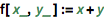`f[x_, y_] := x + y`

Java 程序员备注：

Python 程序员备注：

In:=2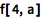`f[4, a]`
Out=2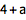In:=3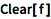`Clear[f]`

In:=1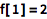`f = 2`
Out=1In:=2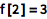`f = 3`
Out=2In:=3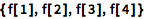`{f, f, f, f}`
Out=3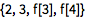In:=4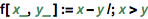`f[x_, y_] := x - y /; x > y`

Java 程序员备注：

Python 程序员备注：

In:=1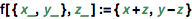`f[{x_, y_}, z_] := {x + z, y - z}`

In:=2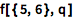`f[{5, 6}, q]`
Out=2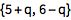Wolfram 语言没有所谓的类型；一切都是符号表达式. 表达式的“标头”可被作为普遍意义上的类型标志符使用.

In:=1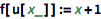`f[u[x_]] := x + 1`

In:=2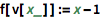`f[v[x_]] := x - 1`

Java 程序员备注：

Python 程序员备注：

Wolfram 语言函数可使用内置的模式匹配来根据其任意参数的符号结构来更改行为. 在 Python 中则需要相对复杂的自定义代码或第三方程序库.